Saturday, March 17, 2012

PHOTOSYNTHESIS LIGHT PHASE ASSIGNMENT

 1 [A]: Carotenoids are made of non-mineral essential elements [R]: Carotenes have C, H and O Both A and R are true and R is the correct explanation of A Both A and R are true but R is not the correct explanation of A A is true but R is false A is false but R is true Ans:A is true but R is false 2 The following pigment is a hydrocarbon. I. Lycopene II. Lutein III. Zeaxanthin IV. β-carotene Only IV II and III I and III I and IV Ans:I and IV 3 Water soluble photosynthetic pigments are seen in Green algae; Red algae Red algae ; Brown algae Blue-green algae ; Red algae Brown algae ; Blue green algae Ans:Blue-green algae ; Red algae 4 [A]: Carotenoids are accessory pigments. [R]: Lycopene protects the chlorophyll from photo-oxidation Both A and R are true and R is the correct explanation of A Both A and R are true but R is not the correct explanation of A A is true but R is false A is false but R is true Ans:Both A and R are true but R is not the correct explanation of A 5 Light Harvesting complex has All pigments except chlorophyll All pigments except phycobilins All pigments except carotenoids All types of photosynthetic pigments Ans:All types of photosynthetic pigments 6 Light energy is converted into chemical energy by Chlorophyll b P 700 Carotene Phycobilin Ans:P 700 7 In photosynthesis the light energy is utilized in the form of Blue light Red light Green light Yellow light Ans:Red light 8 If a LHC absorbs 2000 photons, the minimum number of photons involved in exciting the reaction centre would be 1900 1950 1000 900 Ans:1900 9 The presence of two phases (Light reaction and Dark reaction) in the mechanism of photosynthesis was first reported by Hill Blackman Emerson Ruben Ans:Blackman 10 Match the following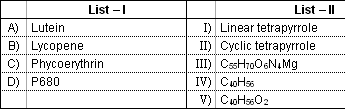A - V, B - III, C - I, D - II A - V, B - IV, C - I, D - II A - IV, B - V, C - II, D - I A - III, B - IV, C - V, D - II Ans:A - V, B - IV, C - I, D - II 11 Study the following table and choose the correct combination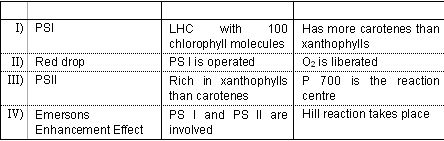I and IV III and IV I and II II and IV Ans:I and IV 12 [A]: NADP has ADP [R]: It has Purine nucleotide Both A and R are true and R is the correct explanation of A Both A and R are true but R is not the correct explanation of A A is true but R is false A is false but R is true Ans:A is false but R is true 13 Potassium ferric oxalate is used to demonstrate Red drop Emerson enhancement effect Role of two Photo systems in photosynthesis Hill reaction Ans:Hill reaction 14 In Z scheme Hill and Bendall did not show The involvement of PSI Translocation of Protons Formation of NADPH Oxidation of P680 Ans:Translocation of Protons 15 [A]: DCMU inhibits non-cyclic electron transport [R]: It prevents the binding of PQ to cytochrome b/f complex Both A and R are true and R is the correct explanation of A Both A and R are true but R is not the correct explanation of A A is true but R is false A is false but R is true Ans:A is true but R is false 16 Number of photons required for the formation of one NADPH during non-cyclic electron transport is 2 4 8 6 Ans:4 17 Choose the incorrect statements I. P680+ is a strong oxidant II. P700+is a strong oxidant III. Energy is lost as heat during the transport of e- from P680 to Pheo IV. The electron that reduces the PC has less energy than the electron that reduces cytochrome b/f complex. All except I All except II All except III All except IV Ans:All except II 18 Arrange the following in a sequence of gradual increase in the amount of energy that they carry. I. P680+ II. PC III. PQH2 IV. P 700 IV, II, III, I IV, III, II, I I, III, II, IV IV, II, I, III Ans:IV, II, III, I 19 The number of photons pumped into lumen from stroma due to oxidation of 12 molecules of water during Z scheme is 24 72 48 12 Ans:48 20 Arrange the following electron carriers in correct sequence of their participation in non-cyclic electron transport. I. PC II. Pheo III. Fd IV. PQ II, I, IV, III II, IV, III, I II, IV, I, III II, I, IV, III Ans:II, IV, I, III 21 Study the following table and choose the correct combination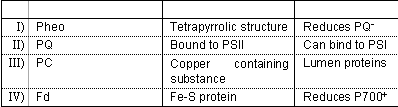I and II II and III III and IV I and III Ans:I and III 22 [A]: P680 is a chlorophyll a molecule with absorption maxima at 680nm [R]: P680 can not absorb blue light Both A and R are true and R is the correct explanation of A Both A and R are true but R is not the correct explanation of A A is true but R is false A is false but R is true Ans:A is true but R is false 23 The following pigment molecule can not transfer the light energy to other pigment pmolecule P700 Chlorophyll a Chlorophyll b Lutein Ans:P700 24 During photosynthesis, the light energy is first converted into Kinetic energy Chemical energy Potential energy Excitation energy Ans:Excitation energy 25 Identify the pigment molecule which has relatively at higher energy level Chl Chl+ Chl* formed due to absorption of blue photon Chl * formed due to absorption of red photon Ans:Chl* formed due to absorption of blue photon 26 [A]: Reaction centre is devoid of chlorophyll b [R]: The photons absorbed by chlorophyll b can not be transferred to reaction centre Both A and R are true and R is the correct explanation of A Both A and R are true but R is not the correct explanation of A A is true but R is false A is false but R is true Ans:A is true but R is false 27 The photons absorbed by antenna pigment molecules is transferred to reaction centre by a phenomenon known as Inductive transformation Transcription Translation Resonance transfer Ans:Resonance transfer 28 [A]: Photosynthetic rate is more in blue light than red light [R]: Blue light has lesser wavelength than red light. Both A and R are true and R is the correct explanation of A Both A and R are true but R is not the correct explanation of A A is true but R is false A is false but R is true Ans:A is false but R is true 29 Match the following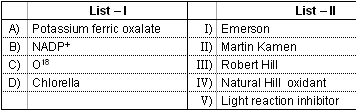A - III, B - IV, C - II, D - I A - IV, B - III, C - II, D - I A - III, B - IV, C - I, D - II A - V, B - IV, C - II, D - I Ans:A - III, B - IV, C - II, D - I 30 [A]: Oxygen is not evolved in bacterial photosynthesis [R]: CO2 is not fixed by photosynthetic bacteria Both A and R are true and R is the correct explanation of A Both A and R are true but R is not the correct explanation of A A is true but R is false A is false but R is true Ans:A is true but R is false 31 Most of the global photosynthesis is carried out by Angiosperms Algae Embryophytes Tracheophytes Ans:Algae 32 During photosynthesis Oxygen is evolved in Stroma Thyloakoid membrane Lumen Chloroplast envelope Ans:Lumen 33 The chemical formula for Chlorophyll b is C55H70O5N4Mg C55H72O5N4Mg C55H70O6N4Mg C55H72O6N4Mg Ans:C55H70O6N4Mg 34 Methyl group of Chlorophyll b is attached to Phytol tail II pyrrole group III pyrrole group IV pyrrole group Ans:II pyrrole group 35 The number of carbon atoms in Phytol tail is 20 35 25 19 Ans:20 36 Chlorophyll b is Olive green Blue green violet green orange green Ans:Olive green 37 Phytol tail of chlorophyll is attached to I pyrrole group II pyrrole grorup III pyrrole group IV pyrrole group Ans:IV pyrrole group 38 Match the following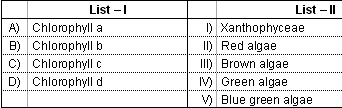A - V, B - IV, C - II, D - I A - I, B - IV, C - III, D - II A - IV, B - V, C - II, D - III A - V, B - IV, C - I, D - III Ans:A - I, B - IV, C - III, D - II 39 [A]: Bacteria do not evolve Oxygen in their photosynthesis [R]: They lack Chlorophylls Both A and R are true and R is the correct explanation of A Both A and R are true but R is not the correct explanation of A A is true but R is false A is false but R is true Ans:A is true but R is false 40 Phytol tail of chlorophyll is esterified to the following pyrrole group of head I IV II III Ans:IV